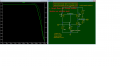# How to get frequency response at LTSPICE?

#### Oppaya

Joined Apr 16, 2021
8I am simulating the Common source amplifier circuit with current mirror. I wanted to see the frequency reponse of this circuit. And I got the constant value as you see the upper graph.I have finally noitced if I change the value the parastic properties at Input Voltage Source, It shows the curve similar as I expected.

I want to ask you I'm on a right way to do AC simulation, And How should I set the parasitic properties. Thank you.

#### Bordodynov

Joined May 20, 2015
3,117
You took a huge capacity of the signal source - that's not right. Standard field-effect transistors have no parasitic capacitance, which gave you a horizontal gain line. You need to take the real models. And the fact that you used resistance and signal source capacitance to simulate the decay is cheating.

•Oppaya

#### Oppaya

Joined Apr 16, 2021
8
You took a huge capacity of the signal source - that's not right. Standard field-effect transistors have no parasitic capacitance, which gave you a horizontal gain line. You need to take the real models. And the fact that you used resistance and signal source capacitance to simulate the decay is cheating.I selected monolithic MOSFET NMOS4, PMOS4, and I downloaded MOSFET models at this websites (http://cmosedu.com/cmos1/book.htm)and included that Models.

And we can see the frequency response like a upper photo.

But, when I have added .model on text and typed DC parameters, It didn't seem to be applied.

And I read that file and didn't find those parameters(kp,kn,Vto,lamba....)

How can I apply it?

• Short channel models from CMOS Circuit Design, Layout, and Simulation,
• 50nm BSIM4 models VDD=1V, see CMOSedu.com
.model N_50n nmos level = 54

+binunit = 1 paramchk= 1 mobmod = 0

+capmod = 2 igcmod = 1 igbmod = 1 geomod = 0

+diomod = 1 rdsmod = 0 rbodymod= 1 rgatemod= 1

+permod = 1 acnqsmod= 0 trnqsmod= 0

+tnom = 27 toxe = 1.4e-009 toxp = 7e-010 toxm = 1.4e-009

+epsrox = 3.9 wint = 5e-009 lint = 1.2e-008

+ll = 0 wl = 0 lln = 1 wln = 1

+lw = 0 ww = 0 lwn = 1 wwn = 1

+lwl = 0 wwl = 0 xpart = 0 toxref = 1.4e-009

+vth0 = 0.22 k1 = 0.35 k2 = 0.05 k3 = 0

+k3b = 0 w0 = 2.5e-006 dvt0 = 2.8 dvt1 = 0.52

+dvt2 = -0.032 dvt0w = 0 dvt1w = 0 dvt2w = 0

+dsub = 2 minv = 0.05 voffl = 0 dvtp0 = 1e-007

+dvtp1 = 0.05 lpe0 = 5.75e-008 lpeb = 2.3e-010 xj = 2e-008

+ngate = 5e+020 ndep = 2.8e+018 nsd = 1e+020 phin = 0

+cdsc = 0.0002 cdscb = 0 cdscd = 0 cit = 0

+voff = -0.15 nfactor = 1.2 eta0 = 0.15 etab = 0

+vfb = -0.55 u0 = 0.032 ua = 1.6e-010 ub = 1.1e-017

+uc = -3e-011 vsat = 1.1e+005 a0 = 2 ags = 1e-020

+a1 = 0 a2 = 1 b0 = -1e-020 b1 = 0

+keta = 0.04 dwg = 0 dwb = 0 pclm = 0.18

+pdiblc1 = 0.028 pdiblc2 = 0.022 pdiblcb = -0.005 drout = 0.45

+pvag = 1e-020 delta = 0.01 pscbe1 = 8.14e+008 pscbe2 = 1e-007

+fprout = 0.2 pdits = 0.2 pditsd = 0.23 pditsl = 2.3e+006

+rsh = 3 rdsw = 150 rsw = 150 rdw = 150

+rdswmin = 0 rdwmin = 0 rswmin = 0 prwg = 0

+prwb = 6.8e-011 wr = 1 alpha0 = 0.074 alpha1 = 0.005

+beta0 = 30 agidl = 0.0002 bgidl = 2.1e+009 cgidl = 0.0002

+egidl = 0.8

+aigbacc = 0.012 bigbacc = 0.0028 cigbacc = 0.002

+nigbacc = 1 aigbinv = 0.014 bigbinv = 0.004 cigbinv = 0.004

+eigbinv = 1.1 nigbinv = 3 aigc = 0.017 bigc = 0.0028

+cigc = 0.002 aigsd = 0.017 bigsd = 0.0028 cigsd = 0.002

+nigc = 1 poxedge = 1 pigcd = 1 ntox = 1

+xrcrg1 = 12 xrcrg2 = 5

+cgso = 6.238e-010 cgdo = 6.238e-010 cgbo = 2.56e-011 cgdl = 2.495e-10

+cgsl = 2.495e-10 ckappas = 0.02 ckappad = 0.02 acde = 1

+moin = 15 noff = 0.9 voffcv = 0.02

+kt1 = -0.21 kt1l = 0.0 kt2 = -0.042 ute = -1.5

+ua1 = 1e-009 ub1 = -3.5e-019 uc1 = 0 prt = 0

+at = 53000

+fnoimod = 1 tnoimod = 0

+jss = 0.0001 jsws = 1e-011 jswgs = 1e-010 njs = 1

+ijthsfwd= 0.01 ijthsrev= 0.001 bvs = 10 xjbvs = 1

+jsd = 0.0001 jswd = 1e-011 jswgd = 1e-010 njd = 1

+ijthdfwd= 0.01 ijthdrev= 0.001 bvd = 10 xjbvd = 1

+pbs = 1 cjs = 0.0005 mjs = 0.5 pbsws = 1

+cjsws = 5e-010 mjsws = 0.33 pbswgs = 1 cjswgs = 5e-010

+mjswgs = 0.33 pbd = 1 cjd = 0.0005 mjd = 0.5

+pbswd = 1 cjswd = 5e-010 mjswd = 0.33 pbswgd = 1

+cjswgd = 5e-010 mjswgd = 0.33 tpb = 0.005 tcj = 0.001

+tpbsw = 0.005 tcjsw = 0.001 tpbswg = 0.005 tcjswg = 0.001

+xtis = 3 xtid = 3

+dmcg = 0e-006 dmci = 0e-006 dmdg = 0e-006 dmcgt = 0e-007

+dwj = 0e-008 xgw = 0e-007 xgl = 0e-008

+rshg = 0.4 gbmin = 1e-010 rbpb = 5 rbpd = 15

+rbps = 15 rbdb = 15 rbsb = 15 ngcon = 1